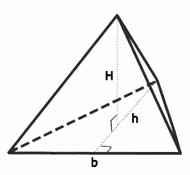# Triangular Pyramid Volume Calculator

With the help of this amazing online calculator, you can calculate the volume of the pyramid, which equals to multiple of its base, triangle height and pyramid ones. You can start calculating the volume of the next pyramid without removing the previous results and refreshing the page. In order to fully understand how the calculator works, read the following instructions.

1. Write down the height of the triangular base (base height).
2. Enter the triangle's base length.
3. Finally, write down a pyramid's height value and click Calculate.

As you see, the only step you need to do in order to use our calculator is to enter all your variables and wait until our calculator has calculated the volume of your pyramid.

Triangular Pyramid Volume Calculator## What is a Pyramid?

According to Merriam-Webster online dictionary, a pyramid is a polyhedron that is formed by combining the polygonal base and a point, which is called the apex.

The calculator computes the volume of a triangular pyramid using the equation below:

• Volume = (Area of Base × Height) ÷ 3
[ Note that the base is triangular, so the area is ½ base × height ]

Note: Do not forget to use the same measurements for different variables while calculating the volume of a particular triangular pyramid.

The volumes of solid objects can be calculated in different measurements, such as cubic feet, cubic meters or cubic yards.

The volumes of liquids as well as of solid objects can be calculated in distinct measurements such as liters, quarts, pints or gallons.

Our online Triangular Pyramid calculator can automatically compute, with the help of the measurements, the volume of the Triangular Pyramid.